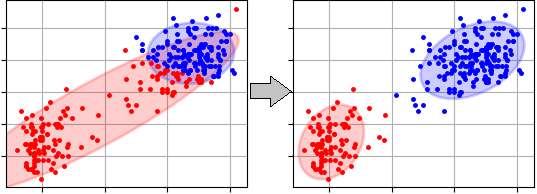pyclustering.cluster.ema.ema Class Reference

Expectation-Maximization clustering algorithm for Gaussian Mixture Model (GMM). More...

## Public Member Functions

def __init__ (self, data, amount_clusters, means=None, variances=None, observer=None, tolerance=0.00001, iterations=100)
Initializes Expectation-Maximization algorithm for cluster analysis. More...

def process (self)
Run clustering process of the algorithm. More...

def get_clusters (self)

def get_centers (self)

def get_covariances (self)

def get_probabilities (self)
Returns 2-dimensional list with belong probability of each object from data to cluster correspondingly, where that first index is for cluster and the second is for point. More...

## Detailed Description

Expectation-Maximization clustering algorithm for Gaussian Mixture Model (GMM).

The algorithm provides only clustering services (unsupervised learning). Here an example of data clustering process:

from pyclustering.cluster.ema import ema, ema_visualizer
from pyclustering.samples.definitions import FCPS_SAMPLES
# Read data from text file.
# Create EM algorithm to allocated four clusters.
ema_instance = ema(sample, 3)
# Run clustering process.
ema_instance.process()
# Get clustering results.
clusters = ema_instance.get_clusters()
covariances = ema_instance.get_covariances()
means = ema_instance.get_centers()
# Visualize obtained clustering results.
ema_visualizer.show_clusters(clusters, sample, covariances, means)

Here is clustering results of the Expectation-Maximization clustering algorithm where popular sample 'OldFaithful' was used. Initial random means and covariances were used in the example. The first step is presented on the left side of the figure and final result (the last step) is on the right side:ema_visualizer
ema_observer

Definition at line 443 of file ema.py.

## ◆ __init__()

 def pyclustering.cluster.ema.ema.__init__ ( self, data, amount_clusters, means = `None`, variances = `None`, observer = `None`, tolerance = `0.00001`, iterations = `100` )

Initializes Expectation-Maximization algorithm for cluster analysis.

Parameters
 [in] data (list): Dataset that should be analysed and where each point (object) is represented by the list of coordinates. [in] amount_clusters (uint): Amount of clusters that should be allocated. [in] means (list): Initial means of clusters (amount of means should be equal to amount of clusters for allocation). If this parameter is 'None' then K-Means algorithm with K-Means++ method will be used for initialization by default. [in] variances (list): Initial cluster variances (or covariances in case of multi-dimensional data). Amount of covariances should be equal to amount of clusters that should be allocated. If this parameter is 'None' then K-Means algorithm with K-Means++ method will be used for initialization by default. [in] observer (ema_observer): Observer for gathering information about clustering process. [in] tolerance (float): Defines stop condition of the algorithm (when difference between current and previous log-likelihood estimation is less then 'tolerance' then clustering is over). [in] iterations (uint): Additional stop condition parameter that defines maximum number of steps that can be performed by the algorithm during clustering process.

Definition at line 480 of file ema.py.

## ◆ get_centers()

 def pyclustering.cluster.ema.ema.get_centers ( self )
Returns
(list) Corresponding centers (means) of clusters.

Definition at line 557 of file ema.py.

## ◆ get_clusters()

 def pyclustering.cluster.ema.ema.get_clusters ( self )
Returns
(list) Allocated clusters where each cluster is represented by list of indexes of points from dataset, for example, two cluster may have following representation [[0, 1, 4], [2, 3, 5, 6]].

Definition at line 548 of file ema.py.

## ◆ get_covariances()

 def pyclustering.cluster.ema.ema.get_covariances ( self )
Returns
(list) Corresponding variances (or covariances in case of multi-dimensional data) of clusters.

Definition at line 566 of file ema.py.

## ◆ get_probabilities()

 def pyclustering.cluster.ema.ema.get_probabilities ( self )

Returns 2-dimensional list with belong probability of each object from data to cluster correspondingly, where that first index is for cluster and the second is for point.

# Get belong probablities
probabilities = ema_instance.get_probabilities();
# Show porbability of the fifth element in the first and in the second cluster
index_point = 5;
print("Probability in the first cluster:", probabilities[index_point]);
print("Probability in the first cluster:", probabilities[index_point]);
Returns
(list) 2-dimensional list with belong probability of each object from data to cluster.

Definition at line 575 of file ema.py.

## ◆ process()

 def pyclustering.cluster.ema.ema.process ( self )

Run clustering process of the algorithm.

This method should be called before call 'get_clusters()'.

Definition at line 521 of file ema.py.

The documentation for this class was generated from the following file: